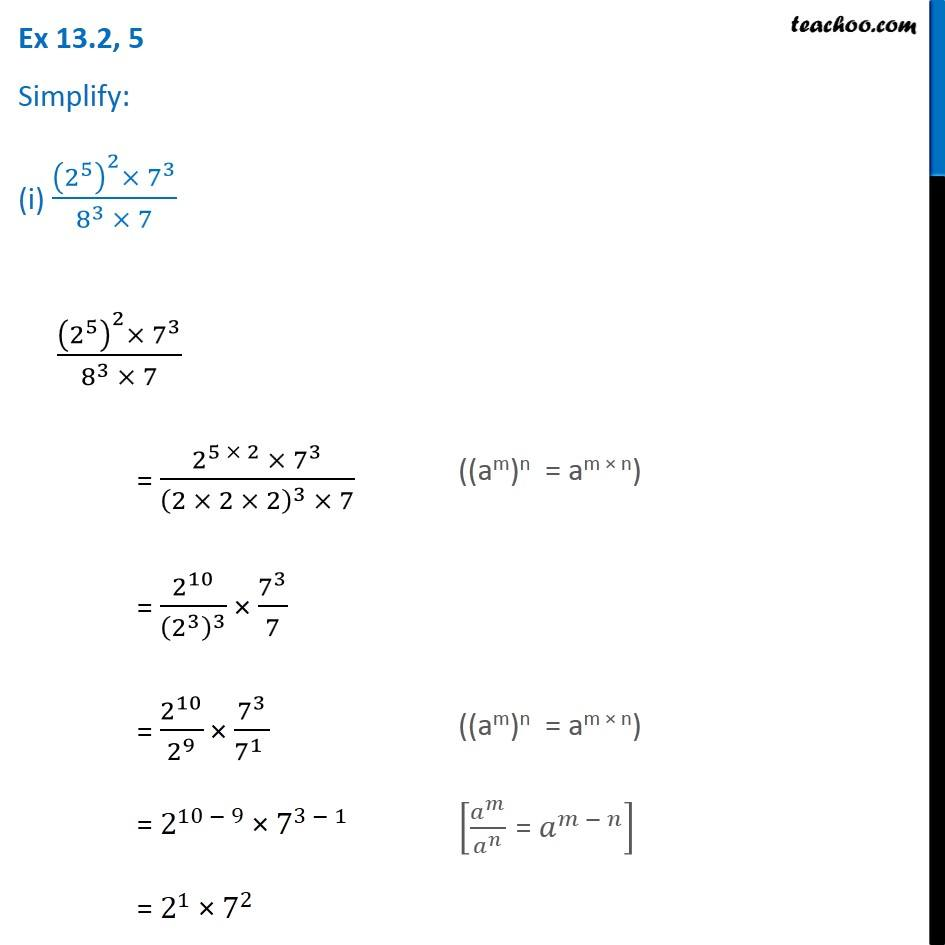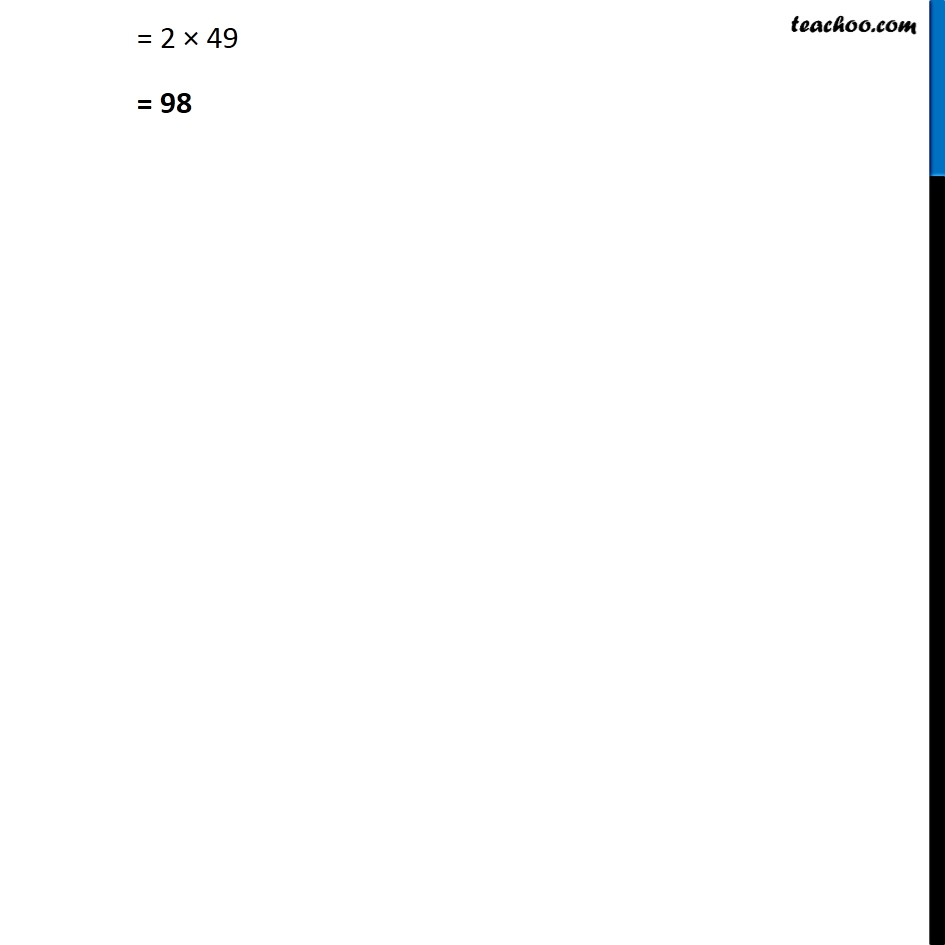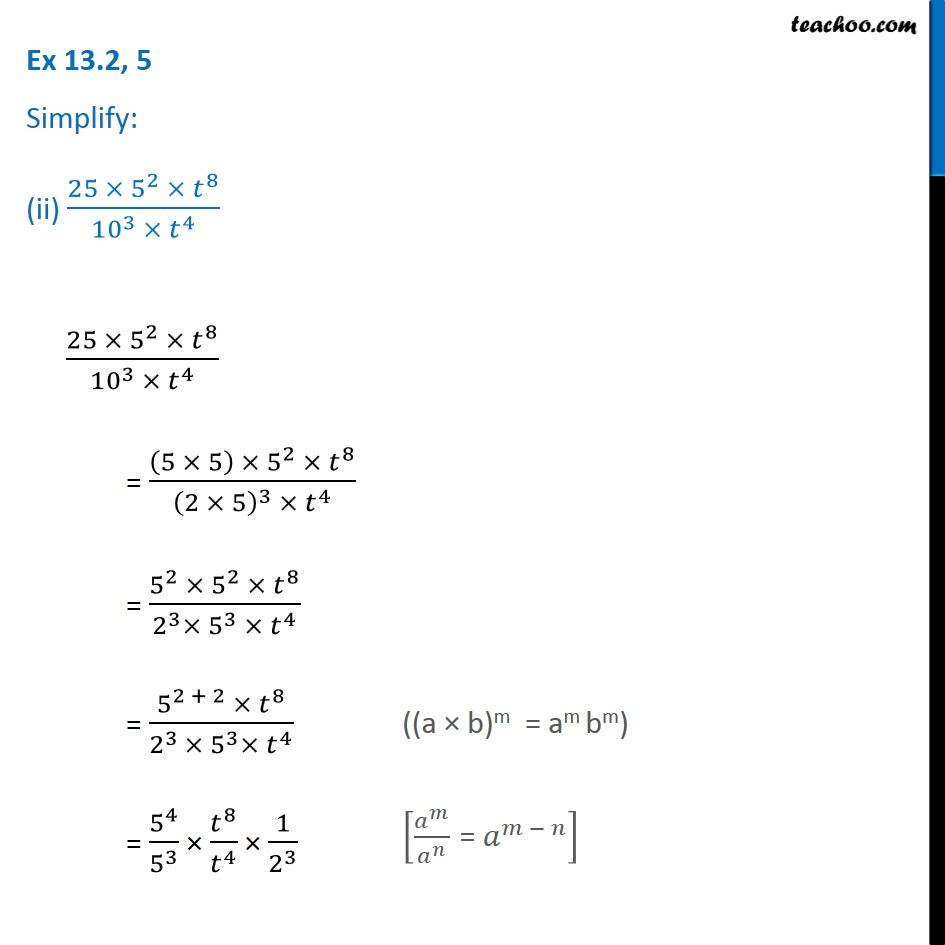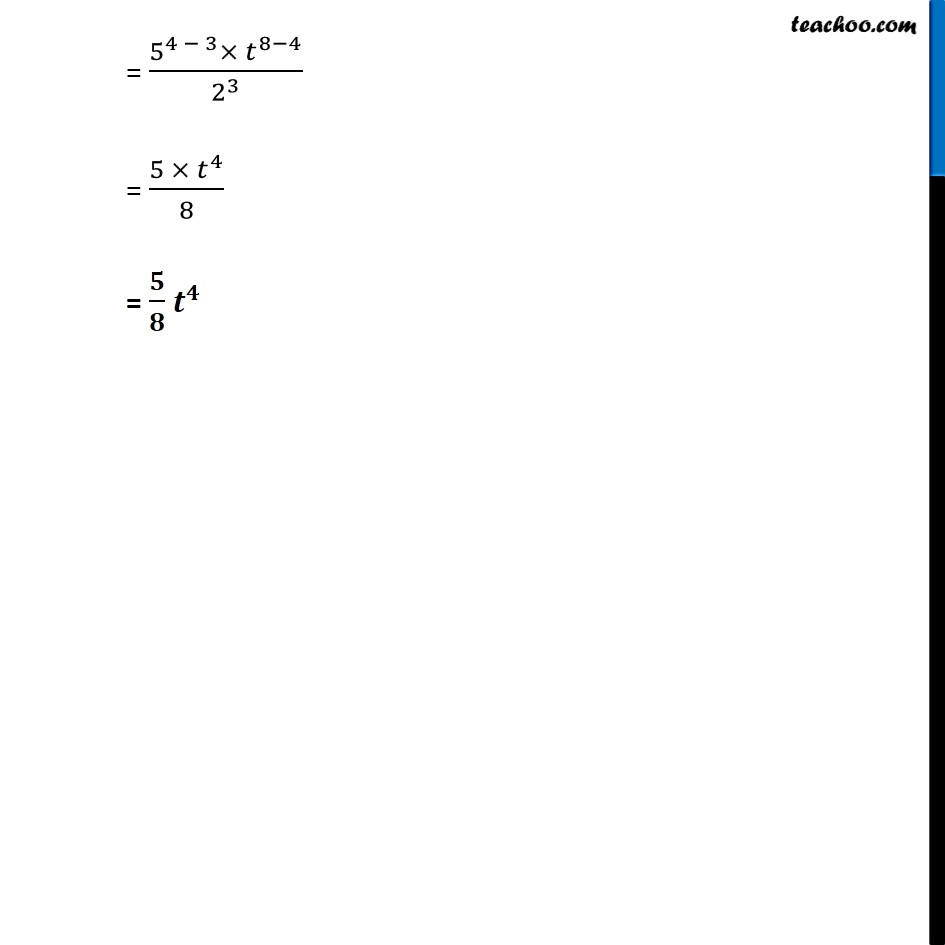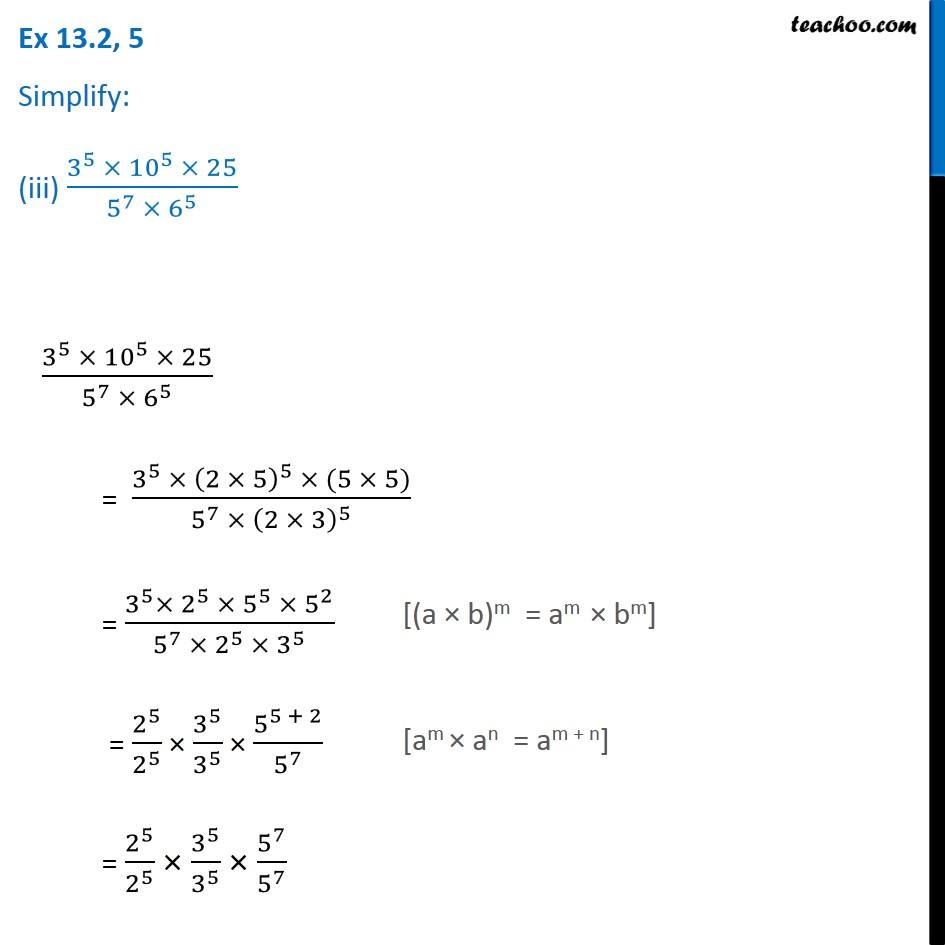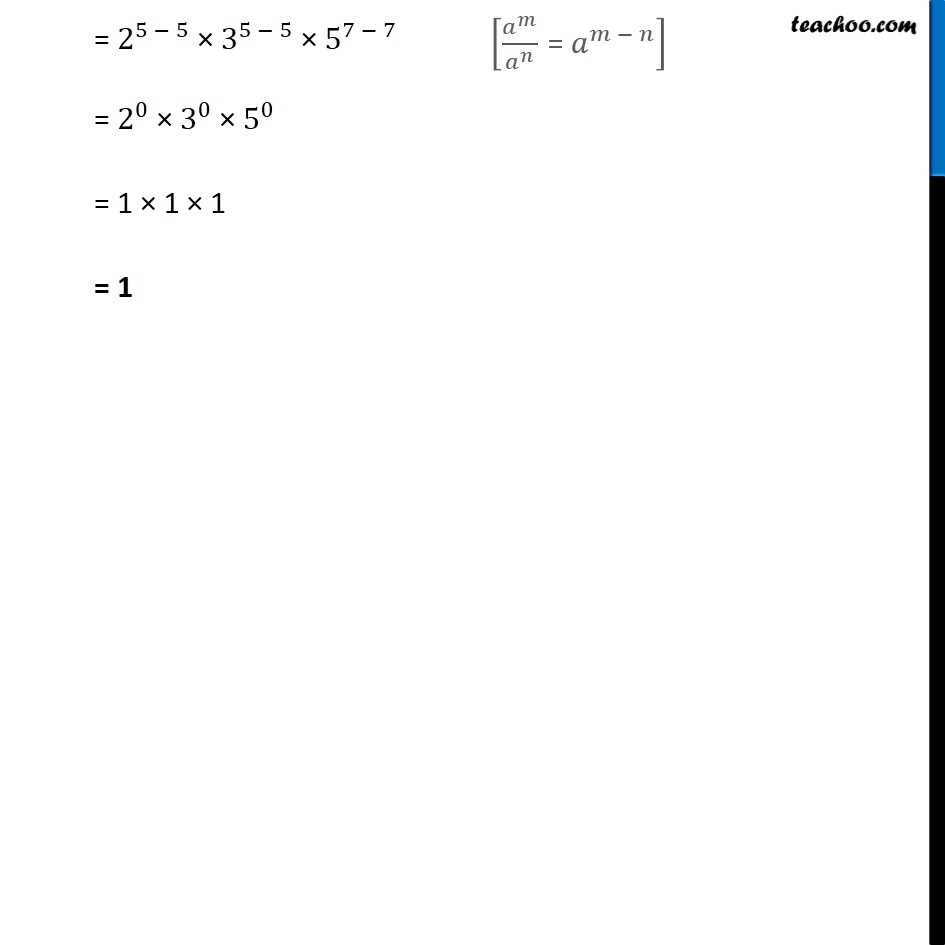1. Chapter 13 Class 7 Exponents and Powers
2. Serial order wise
3. Ex 13.2

Transcript

Ex 13.2, 5 Simplify: (i) ((2^5 )^2×〖 7〗^3)/(8^3 × 7) Ex 13.2, 5 Simplify: (i) ((2^5 )^2×〖 7〗^3)/(8^3 × 7) ((am)n = am × n) = 2^10/(2^3 )^3 × 7^3/7 ((am)n = am × n) = 2^(10 − 9) × 7^(3 − 1) [𝑎^𝑚/𝑎^𝑛 " = " 𝑎^(𝑚 − 𝑛) ] = 2^1 × 7^2 = 2 × 49 = 98 Ex 13.2, 5 Simplify: (ii) (25 ×〖 5〗^2 ×〖 𝑡〗^8)/(〖10〗^3 ×〖 𝑡〗^4 ) (25 ×〖 5〗^2 ×〖 𝑡〗^8)/(〖10〗^3 ×〖 𝑡〗^4 ) = ((5 × 5) × 5^2 × 𝑡^8)/((2 × 5)^3 × 𝑡^4 ) = (5^2 × 5^2 × 𝑡^8)/(2^3× 5^3 ×〖 𝑡〗^4 ) = (5^(2 + 2) ×〖 𝑡〗^8)/(2^(3 )× 5^3× 𝑡^4 ) = 5^4/5^3 × 𝑡^8/𝑡^4 × 1/2^3 ((a × b)m = am bm) [𝑎^𝑚/𝑎^𝑛 " = " 𝑎^(𝑚 − 𝑛) ] = (5^(4 − 3)× 𝑡^(8−4))/2^3 = (5 × 𝑡^4)/8 = 𝟓/𝟖 𝒕^𝟒 Ex 13.2, 5 Simplify: (iii) (3^5 × 〖10〗^5 × 25)/(5^7 ×〖 6〗^5 ) (3^5 × 〖10〗^5 × 25)/(5^7 ×〖 6〗^5 ) = (3^5 × (2 × 5)^5 × (5 × 5))/(5^7 × (2 × 3)^5 ) = (3^5× 2^5 × 5^5 × 5^2)/(5^7 ×〖 2〗^5 × 3^5 ) = 2^5/2^5 × 3^5/3^5 × 5^(5 + 2)/5^7 = 2^5/2^5 × 3^5/3^5 × 5^7/5^7 [(a × b)m = am × bm] [am × an = am + n] = 2^(5 − 5) × 3^(5 − 5) × 5^(7 − 7) = 2^0 × 3^0 × 5^0 = 1 × 1 × 1 = 1 [𝑎^𝑚/𝑎^𝑛 " = " 𝑎^(𝑚 − 𝑛) ]

Ex 13.2